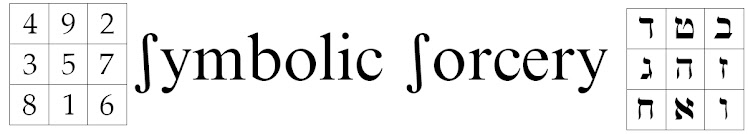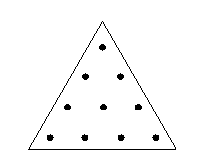## Tuesday, August 2, 2011

### Some thoughts on Mathematical Alchemy

See:Hear:

The 4th of the 32 Paths is called the Measuring Intelligence. In Key 4 we see the Emperor weighing the sphere in the left hand, and holding a measuring tool in the right hand. Heh is the Window, HH = 10. There are 10 points in the Pythagorean Tetraktys, which is our philosophical counting tool. 1+2+3+4 = 10 = 1

Listen:

"The Pythagoreans considered all mathematical science to be divided into four parts: one half they marked off as concerned with quantity, the other half with magnitude; and each of these they posited as twofold. A quantity can be considered in regard to its character by itself or in its relation to another quantity; magnitudes as either stationary or in motion. Arithmetic, then, studies quantity as such; music the relations between quantities; geometry [studies] magnitude at rest, spherics [studies] magnitude inherently moving."

-- Proclus: A Commentary on the First Book of Euclid's Elements

Clarity:

The Via Mathesis is the path of spiritual attainment through the inner qualitative experience of quantitative mathematical truth. The basic skills of the mathematical alchemist are weighing and counting, reminding us of the a Masonic doctrine that "the Master Builder hath ordered all things by weight, measure, and number."